## NCERT Solutions for Class 10 Science Chapter 12 Electricity

These Solutions are part of NCERT Solutions for Class 10 Science. Here we have given NCERT Solutions for Class 10 Science Chapter 12 Electricity. LearnInsta.com provides you the Free PDF download of NCERT Solutions for Class 10 Science (Physics) Chapter 12 – Electricity solved by Expert Teachers as per NCERT (CBSE) Book guidelines. All Chapter 12 – Electricity Exercise Questions with Solutions to help you to revise complete Syllabus and Score More marks. Register for our free webinar class with best Science tutor in India.

NCERT Questions

In Text Questions

Question 1.
What does an electric circuit mean ? (CBSE 2011, 2013, 2014)
An electric circuit is a closed conducting path containing a source of potential difference or electric energy (i.e. a cell or battery) and a device or element utilizing the electric energy.

More Resources

Question 2.
Define the unit of current. (CBSE 2008)
Unit of electric current is ampere. Electric current in a conductor is said to be 1 A if 1 coulomb charge flows through the cross-section of the conductor in 1 second.

Question 3.
Calculate the number of electrons consisting one coulomb of charge. (CBSE 2015)Question 4.
Name a device that helps to maintain a potential difference across a conductor. (CBSE 2008)
A cell or battery.

Question 5.
What is meant by saying that a potential difference between two points is 1 V ?
[CBSE (Delhi) 2008 ; CBSE (All India) 2008, 2010 Term I]
Or
Define the term “Volt”. (CBSE 2009, 2013)
Potential difference between two points is 1 V if 1 joule work is done in moving 1 coulomb charge from one point to another point.

Question 6.
How much energy is given to each coulomb of charge passing through a 6 V battery ?
Energy = Charge x Potential difference =1 C X 6 V = 6 J.

Question 7.
On what factors does the resistance of a conductor depend ? (CBSE 2009, 2011, 2012, 2013, 2014)
Resistance of a conductor depends on

1. length (l) of the conductors,
2. area of corss-section of the conductor,
3. nature of the material of the conductor and
4. temperature of the conductor.

Question 8.
Will current flow more easily through a thick wire or a thin wire of the same material, when connected to the same source Why ?
Area of cross-sectiontherefore, resistance of thin wire is more than the resistance of thick wire. Hence, current in thick wire flows easily than in thin wire.
Let the resistance of an electrical component remains constant while the potential difference across the ends of the component decreases to half of its former value.

Question 9.
What change will occur with current through it ?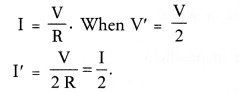Thus, current in the component becomes half of its former value.

Question 10.
Why are coil of electric toasters and electric irons made of an alloy rather than a pure metal ?
(CBSE 2011, 2012)
This is because the resistivity of an alloy is more than the resistivity of a pure metal and hence more heat is produced in an alloy than in pure metal due to the flow of current. Moreover, alloy does not burn (or oxidise) easily even at higher temperature.

Question 11.
(a) Which among, iron and mercury is a better conductor ? (resistivity of iron = 10.0 x 10-8 Ω m and resistivity of mercury = 94 x 10-8 Ω m)
(b) Which material is the best conductor ?
(a) A material whose resistivity is low is a good conductor of electricity. Therefore, iron is better conductor than mercury.
(b) Silver is the best conductor of electricity.

Question 12.
Draw a schematic diagram of a circuit consisting of a batteries of three of 2 V each, a 5 Ω resistor, 8 Ω resistor and a 12 Ω resistor and a plug key, all connected in series. (CBSE 2011)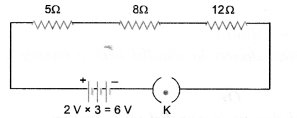Question 13.
Redraw the circuit of question 12, putting an ammeter to measure the current through the resistor and a voltmeter to measure the potential difference across 12 Ω resistor. What would be the reading in the ammeter ?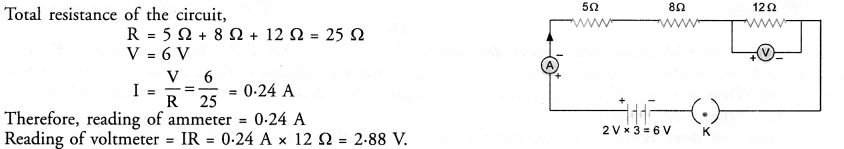Question 14.
Judge the equivalent resistance when the following are connected in parallel :
(a) 1 Ω and 106 Ω
(b) 1 Ω and 103 Ω and 106 Ω.
(CBSE 2013)
(a) When resistors are connected in parallel, then equivalent resistance of the combination is less than the least resistance in the combination. Therefore, equivalent resistance of 1 Ω and 106 Ω connected in parallel is approximately 1 Ω but less than 1 Ω.
(b) The equivalent resistance is approximately 1 Ω but less than 1 Ω.

Question 15.
An electric lamp of 100 W, a toaster of resistance 50 Ω, and a water filter of resistance 500 Ω are connected in parallel to 220 V source. What is the resistance of an electric iron connected to the same source that takes as much current as all three appliances and what is the current through it ?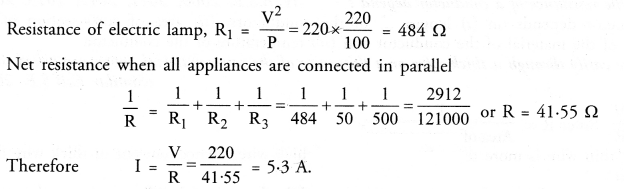Question 16.
What is
(a) highest
(b) lowest resistance that can be secured by combining four coils of resistances 4 Ω, 8 Ω, 12 Ω, 24 Ω ? (CBSE 2010 Term I, 2012, 2013)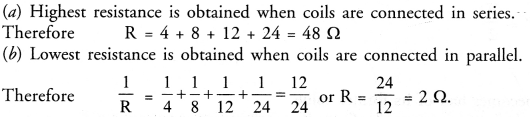Question 17.
Why does the connecting cord of an electric heater not glow while the heating element does ? [CBSE (Delhi) 2008, 2010, 2011, 2013]
This is because resistance of cord of electric heater is less than the resistance of heating element. So more heat is produced in the heating element and less heat is produced in the cord. Due to more heat, heating element glows.

Question 18.
Compute the heat generated while transferring 96000 coulomb of charge in one hour through a potential difference of 50 V. (CBSE 2012, 2013)Question 19.
An electric iron of resistance 20 Ω takes a current of 5 A. Calculate the heat developed in 30 seconds. (CBSE 2010)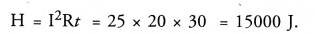Question 20.
What are the advantages of connecting electrical devices in parallel with a battery instead of connecting them in series ?
Or
Give two advantages of connecting electrical devices in parallel with battery. (CBSE 2010 Term I)
Or
In a house-hold electric circuit different appliances are connected in parallel to one another. Give two reasons. (CBSE Sample Papers, CBSE 2012)
Or
Why is parallel arrangement used in domestic ? (NCERT Question Bank, CBSE 2015)

1. If any one of the electric devices in parallel fuses, then the working of other devices will not be affected.
2. When different devices are connected in parallel, they draw the current as per their requirement and hence they work properly.

Question 21.
How can three resistors of resistances 2 Ω, 3 Ω and 6 Ω be connected to give a total resistance of
(a) 4 Ω
(b) 1 Ω ? (CBSE 2012)
(a) We get 4 Ω resistance if 3 Ω and 6 Ω resistors are connected in parallel and this parallel combination is . connected in series with 2 Ω as shown in figure.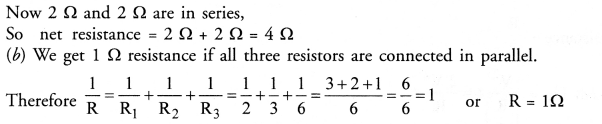Question 22.
What determines the rate at which energy is delivered by an electric current ? [CBSE (All India) 2008]
Electric power determines the rate at which energy is delivered by an electric current.

Question 23.
An electric motor takes 5 A from a 220 V line. Determine the power of the motor and energy consumed in 2 h.
[CBSE (All India) 2008]
Power, P = VI = 220 x 5 = 1100 W
Energy consumed = Power x Time
= 1100 W x 2 h = 2200 Wh = 2.2 kWh.

NCERT Chapter End Exercises

Question 1.
A piece of wire of resistance R is cut into five equal parts. These parts are then connected in parallel. If the equivalent resistance of this combination is R’, then the ratio R/R’ is
(a) 1/25
(b) 1/5
(c) 5
(d) 25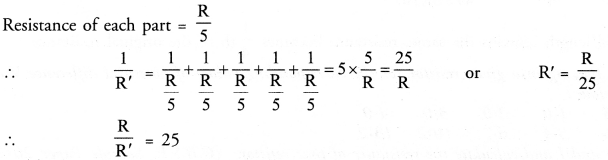Question 2.
Which of the following terms does not represent electrical power in a circuit ?
(a) I2R
(b) IR2
(c) VI
(d) V2/R

Question 3.
An electric bulb is rated as 220 V and 100 W. When it is operated on 110 V the power consumed will be
(a) 100 W
(b) 75 W
(c) 50 W
(d) 25 W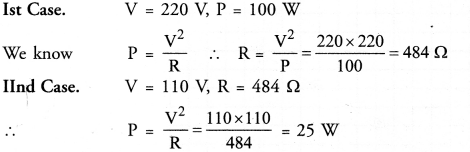∴ (d) is the correct answer.

Question 4.
Two conducting wires of the same material and of equal lengths and equal diameters are first connected in series and then parallel in an electric circuit. The ratio of heat produced in series and parallel combinations would be
(a) 1 :2
(b) 2 : 1
(c) 1 : 4
(d) 4 : 1.Question 5.
How is voltmeter connected in circuit to measure the potential difference between two points ? (CBSE 2011, 2012)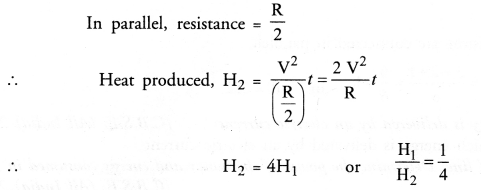Question 6.
A copper wire has diameter 0.5 mm and resistivity of 1.6 x 10-8  Ω m. What will be the length of this wire to make its resistance 10 Ω ? How much does the resistance change if diameter is doubled ?When D is doubled and length remains the same, resistance becomes 1/4 th of the original resistance.

Question 7.
The values of current I flowing in a given resistor for the corresponding values of potential difference V across the resistor are given below :Plot a graph between V and I and calculate the resistance of that resistor. (CBSE Sample Paper 2017-18)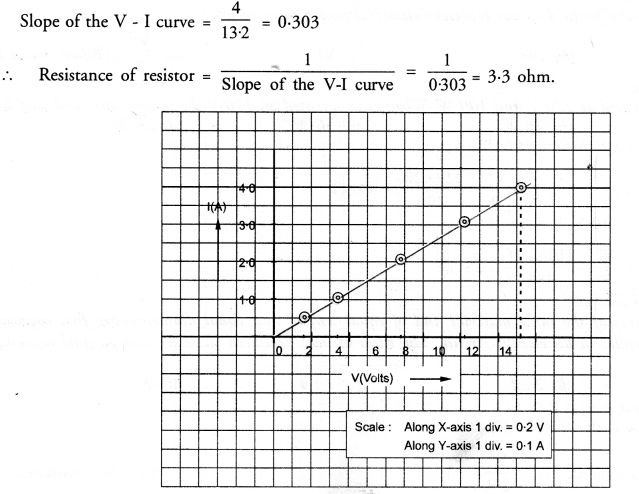Question 8.
When a 12 V battery is connected across an unknown resistance, there is a current of 2.5 mA in the circuit. Find the value of resistance of the resistor.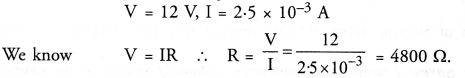Question 9.
A battery of 9 V is connected in series with resistors of 0.2  Ω, 0.3  Ω, 0.4  Ω, 0.5  Ω and 12  Ω respectively. How much current would flow through 12 Ω resistor ? (CBSE 2010 Term I)Question 10.
How many 176  Ω resistors (in parallel) are required to carry 5 A on 220 V line ?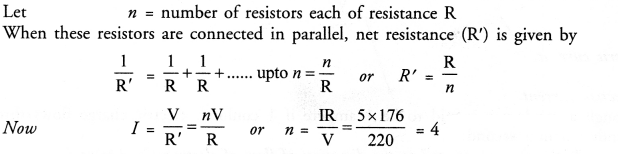Practical Skills Based Questions (Two Marks Questions)

Question 1.
In,a voltmeter, there are 20 divisions between the 0 mark and 0.5 mark. Calculate the least count of the voltmeter.
(CBSE 2015)
Least count of voltmeter= value of 1 division
Here, 20 divisions = 0.5 V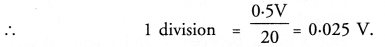Thus, least count of voltmeter = 0.025 V.

Question 2.
To verify Ohm’s law in the laboratory. Name the following circuit components used. (CBSE 2015)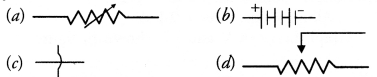(a) Variable resistance
(b) Battery
(c) Wire crossing without joining

Question 3.
A student prepared a circuit diagram to study the dependence of potential difference (V) on electric current (I) across a resistor.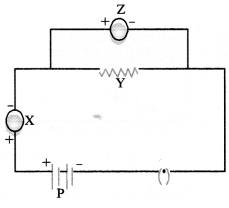In this circuit diagram X, Y, Z, P are represented for the components.
(a) X = ……………..
(b) Y = ……………..
(c) Z = ………………
(d) P = ………………
(CBSE 2015)
X = Ammeter
Y = Resistor
Z = Voltmeter
P = Battery.

Question 4.
Least count of a voltmeter and an ammeter in given figures would respectively be : (CBSE 2015)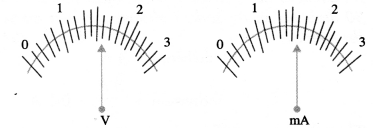Least count of a voltmeter or an ammeter is equal to the value of 1 division on the scale.
In a voltmeter, 5 divisions = IV
or 1 division = 1/5 V = 0.2 V
Thus, least count of voltmeter = 0.2 mA
In an ammeter, 5 division = 1 mA
or 1 division = 1/5 mA = 0.2 mA
Thus, least count of ammeter = 0.2 mA.

Question 5.
To verify Ohm’s law, a student drew a circuit diagram which is given below :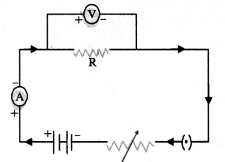1. Name two components in this circuit diagram which are connected in series.
2. Name two components in this circuit diagram which are connected in parallel. (C. B. S. E. 2015)

1. Key, rhoestat, battery, ammeter and resistor are connected in series.
2. Resistor of resistance R and voltmeter (V) are connected in parallel.

Question 6.
In the Ohm’s law experiment, it is advised to take out the key from the plug when the observations are not being taken. Why it is essential l (CBSE 2015)
Ohm’s law is valid only if the resistance of a resistor remains constant ‘during the experiment. If the key from the plug is not taken out when the observations are not taken, then the resistance of the resistor increases due to heat produced as a result of continuous flow of current through it. Hence, Ohm’s law cannot be verified.

Question 7.
The rest positions of the pointers of a milliammeter and volt-meter not in use are as shown in fig. A. When a student uses these in his experiment, the reading of pointers are in positions shown in fig. B.Calculate the corrected value of current and voltage in this experiment.  (CBSE 2015)
Least count of milliammeter = 10mA/5 = 2 mA
Least count of voltmeter = 1V/5 = 0.2 V
Zero error of milliammeter = 2 x 2 mA = 4 mA
Zero error of voltmeter = 3 x 0.2 V = 0.6 V
Correct value of current = 38 mA + 4 mA = 42 mA
Correct value of voltmeter = 3.6V – 0.6 V = 3.0 V.

Question 8.
In an experiment to study the dependence of current on potential difference across a resistor, a student obtained a graph as shown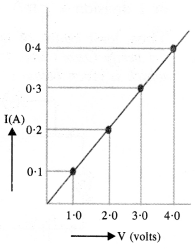Calculate the value of resistance of the resistor. (CBSE 2015)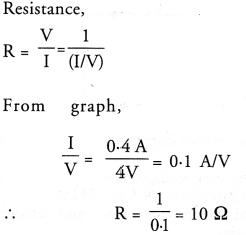Question 9.
In a given ammeter, a student sees that needle indicates 17 divisions in ammeter while performing an experiment to verify Ohm’s law. If ammeter has 10 divisions between 0 and 0.5 A, then what is the value corresponding to 17 divisions ?  (CBSE Sample Paper 2017-18)
10 Div = 0.5 A
1 Div = 0.5 A/10 = 0.05 A
17 Div = 0.05 A x 17 = 0.85 A

Question 10.
While performing an experiment to verify Ohm’s law, what precautions are to be taken ?

1. Connections should be tight.
2. The conductor used should be such that its resistance does not change much with increase in temperature.
3. The plug of the key must only be inserted while reading ammeter and voltmeter. There after, the plug of the key must be taken out to avoid heating of conductor with the continuous flow of current through it.

Question 11.
In a given voltmeter, a student sees that the needle indicates 12 divisions in voltmeter while performing an experiment to verify Ohm s law. If voltmeter has 10 divisions between 0 and 1.0 V, then what is the value corresponding to 12 divisions ?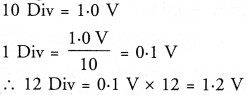Question 12.
The values of current I flowing in a given resistor for the corresponding values of potential difference V across the resistor are given below :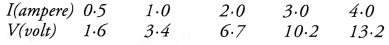Plot a graph between V and I and calculate the resistance of the resistor. (CBSE Sample Paper 2017-18)
Scale : Along x-axis, 1 div = 0.1 V
Along y-axis, 1 div = 0.1 A
Graph between V and I is shown in figure: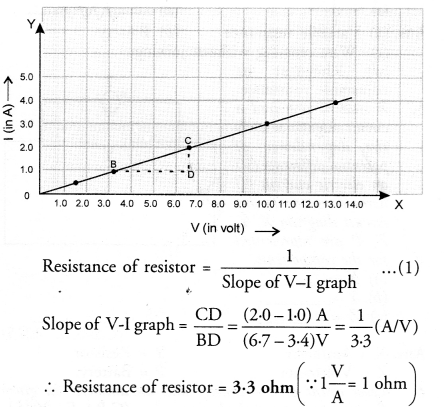Question 13.
An ammeter has a range of 0—3 ampere and there are 30 divisions on the scale. Calculate the least count of the ammeter. (CBSE 2015)